Share

# Construct Triangleabc with Bc = 7.5 Cm, Ac = 5 Cm and M∠C = 60°. - CBSE Class 7 - Mathematics

ConceptConstructing a Triangle When the Lengths of Two Sides and the Measure of the Angle Between Them Are Known. (Sas Criterion)

#### Question

Construct ΔABC with BC = 7.5 cm, AC = 5 cm and m∠C = 60°.

#### Solution

A rough sketch of the required triangle is as follows.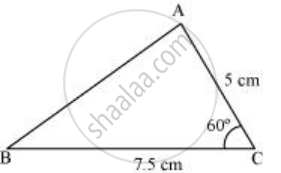The steps of construction are as follows.

1) Draw a line segment BC of length 7.5 cm.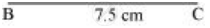2) At point C, draw a ray CX making 60º with BC.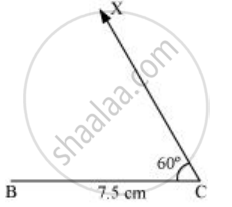3) Taking C as centre, draw an arc of 5 cm radius. It intersects CX at point A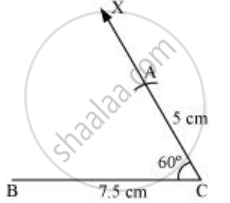4) Join A to B to obtain triangle ABC.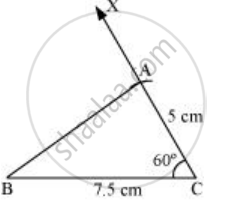Is there an error in this question or solution?

#### APPEARS IN

NCERT Solution for Mathematics for Class 7 (2018 to Current)
Chapter 10: Practical Geometry
Ex. 10.30 | Q: 3 | Page no. 200
Solution Construct Triangleabc with Bc = 7.5 Cm, Ac = 5 Cm and M∠C = 60°. Concept: Constructing a Triangle When the Lengths of Two Sides and the Measure of the Angle Between Them Are Known. (Sas Criterion).
S